# 14-240/Tutorial-October14

## Boris

#### Elementary and (Not So Elementary) Errors in Homework

Consider these three matrices: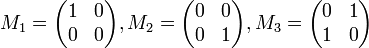$M_1 = \begin{pmatrix} 1 & 0 \\ 0 & 0 \end{pmatrix}, M_2 = \begin{pmatrix} 0 & 0 \\ 0 & 1 \\ \end{pmatrix}, M_3 = \begin{pmatrix} 0 & 1 \\ 1 & 0 \\ \end{pmatrix}$

We want to equate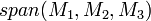$span(M_1, M_2, M_3)$ to the set of all symmetric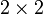$2 \times 2$ matrices. Here is the wrong way to write this: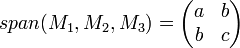$span(M_1, M_2, M_3) = \begin{pmatrix} a & b \\ b & c \\ \end{pmatrix}$.

Firstly,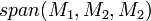$span(M_1, M_2, M_2)$ is the set of all linear combinations of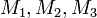$M_1, M_2, M_3$. To equate it to a single symmetric$2 \times 2$ matrix makes no sense. Secondly, the elements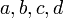$a, b, c, d$ are undefined. What are they suppose to represent? Rational numbers? Real numbers? Members of the field of two elements? The following way of writing erases those issues: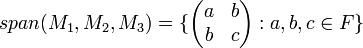$span(M_1, M_2, M_3) = \{ \begin{pmatrix} a & b \\ b & c \\ \end{pmatrix} :a, b, c \in F \}$ where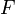$F$ is an arbitrary field.

(2) Algorithm vs. Proof (Boris's Section Only)

When solving a problem that requires a solution to a linear equation, it is not always obvious which of the following you should show:

a) An algorithm for finding the solution
b) A proof that a solution is correct

If the problem asks to solve a linear equation, then just show (a). Otherwise, consider problems such as this:

Determine if the vector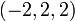$(-2, 2, 2)$ is a linear combination of the vectors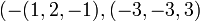$(- (1, 2, -1), (-3, -3, 3)$ in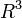$R^3$.

Show both (a) and (b) to be on the safe side.

#### Problem 5h) on Page 34 in Homework 3 for all Fields

For an arbitrary field$F$, determine if the matrix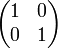$\begin{pmatrix} 1 & 0 \\ 0 & 1 \\ \end{pmatrix}$ is in span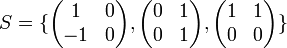$S=\{ \begin{pmatrix} 1 & 0 \\ -1 & 0 \\ \end{pmatrix}, \begin{pmatrix} 0 & 1 \\ 0 & 1 \\ \end{pmatrix}, \begin{pmatrix} 1 & 1 \\ 0 & 0 \\ \end{pmatrix} \}$.

Proof:

We show that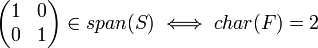$\begin{pmatrix} 1 & 0 \\ 0 & 1 \\ \end{pmatrix} \in span(S) \iff char(F)=2$.

We show that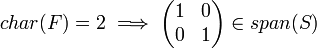$char(F)=2 \implies \begin{pmatrix} 1 & 0 \\ 0 & 1 \\ \end{pmatrix} \in span(S)$.
Assume that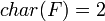$char(F)=2$.
Let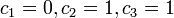$c_1=0, c_2=1, c_3=1$.
Then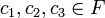$c_1, c_2, c_3 \in F$.
Then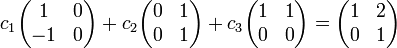$c_1 \begin{pmatrix} 1 & 0 \\ -1 & 0 \\ \end{pmatrix} + c_2 \begin{pmatrix} 0 & 1 \\ 0 & 1 \\ \end{pmatrix} + c_3 \begin{pmatrix} 1 & 1 \\ 0 & 0 \\ \end{pmatrix} = \begin{pmatrix} 1 & 2 \\ 0 & 1 \\ \end{pmatrix}$.
Since$char(F)=2$ and the entries of the matrix are from$F$, then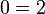$0=2$.
Then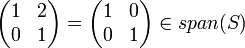$\begin{pmatrix} 1 & 2 \\ 0 & 1 \\ \end{pmatrix} = \begin{pmatrix} 1 & 0 \\ 0 & 1 \\ \end{pmatrix} \in span(S)$.
Then$char(F)=2 \implies \begin{pmatrix} 1 & 0 \\ 0 & 1 \\ \end{pmatrix} \in span(S)$.
We show that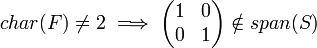$char(F) \neq 2 \implies \begin{pmatrix} 1 & 0 \\ 0 & 1 \\ \end{pmatrix} \notin span(S)$.
Assume to the contrary that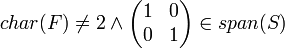$char(F) \neq 2 \and \begin{pmatrix} 1 & 0 \\ 0 & 1 \\ \end{pmatrix} \in span(S)$.
Then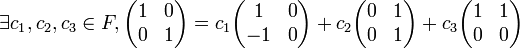$\exists c_1, c_2, c_3 \in F, \begin{pmatrix} 1 & 0 \\ 0 & 1 \\ \end{pmatrix} =c_1 \begin{pmatrix} 1 & 0 \\ -1 & 0 \\ \end{pmatrix} +c_2 \begin{pmatrix} 0 & 1 \\ 0 & 1 \\ \end{pmatrix} +c_3 \begin{pmatrix} 1 & 1 \\ 0 & 0 \\ \end{pmatrix}$.
Then this system of linear equations has a solution: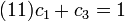$(11)c_1+c_3=1$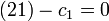$(21)-c_1=0$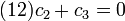$(12)c_2+c_3=0$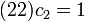$(22)c_2=1$.
When solving this system, we see that it has no solution.
This contradicts the assumption that it has a solution.
Then$char(F) \neq2 \implies \begin{pmatrix} 1 & 0 \\ 0 & 1 \\ \end{pmatrix} \notin span(S)$.
Then$\begin{pmatrix} 1 & 0 \\ 0 & 1 \\ \end{pmatrix} \in span(S) \iff char(F)=2$. Q.E.D.

#### A Field Problem

Find the solution to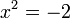$x^2 = -2$ in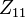$Z_{11}$.

Note that a polynomial of$n$ degree has at most$n$ solutions.

Algorithm:

We find the solution to$x^2 = -2$ in$Z_{11}$.

Since in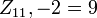$Z_{11}, -2 = 9$, then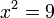$x^2 = 9$.
Since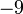$-9$ is additive inverse of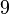$9$, then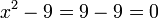$x^2 - 9 = 9 - 9 = 0$.
By the result that we proved in Question 2 of Homework 1, then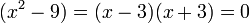$(x^2 - 9) = (x - 3)(x + 3) = 0$.
Then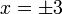$x = \pm 3$ are the solutions.

#### A Dimension Problem

Let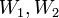$W_1, W_2$ be subspaces of a finite dimensional vector space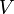$V$ over a field$F$ where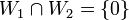$W_1 \cap W_2 = \{0\}$. Then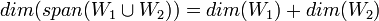$dim(span(W_1 \cup W_2)) = dim(W_1) + dim(W_2)$.

Proof:

We show that$dim(span(W_1 \cup W_2)) = dim(W_1) + dim(W_2)$.

Since$V$ is finite dimensional, then$W_1, W_2$ are finite dimensional.
Then we can let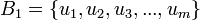$B_1 = \{u_1, u_2, u_3, ..., u_m\}$ be a basis of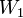$W_1$ and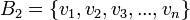$B_2 = \{v_1, v_2, v_3, ..., v_n\}$ be a basis of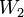$W_2$.
We show that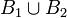$B_1 \cup B_2$ is a basis of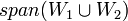$span(W_1 \cup W_2)$.
We show that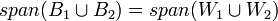$span(B_1 \cup B_2) = span(W_1 \cup W_2)$.
We show that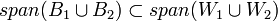$span(B_1 \cup B_2) \subset span(W_1 \cup W_2)$.
Since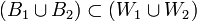$(B_1 \cup B_2) \subset (W_1 \cup W_2)$, then$span(B_1 \cup B_2) \subset span(W_1 \cup W_2)$.
We show that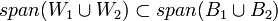$span(W_1 \cup W_2) \subset span(B_1 \cup B_2)$.
Since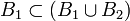$B_1 \subset (B_1 \cup B_2)$, then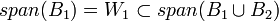$span(B_1) = W_1 \subset span(B_1 \cup B_2)$.
Since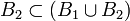$B_2 \subset (B_1 \cup B_2)$, then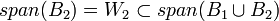$span(B_2) = W_2 \subset span(B_1 \cup B_2)$.
Then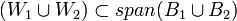$(W_1 \cup W_2) \subset span(B_1 \cup B_2)$ and$span(W_1 \cup W_2) \subset span(B_1 \cup B_2)$.
Then$span(B_1 \cup B_2) = span(W_1 \cup W_2)$.
We show that$B_1 \cup B_2$ is linearly independent.
Let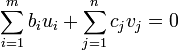$\displaystyle\sum_{i=1}^{m} b_iu_i + \displaystyle\sum_{j=1}^{n} c_jv_j = 0$ where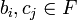$b_i, c_j \in F$.
Then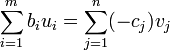$\displaystyle\sum_{i=1}^{m} b_iu_i = \displaystyle\sum_{j=1}^{n} (-c_j)v_j$.
Since$W_1 \cap W_2 = \{ 0 \}$, then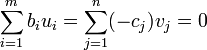$\displaystyle\sum_{i=1}^{m} b_iu_i = \displaystyle\sum_{j=1}^{n} (-c_j)v_j = 0$.
Since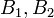$B_1, B_2$ are linearly independent, then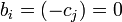$b_i = (-c_j) = 0$.
For$\displaystyle\sum_{i=1}^{m} b_iu_i + \displaystyle\sum_{j=1}^{n} c_jv_j = 0$, then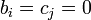$b_i = c_j = 0$.
Then$B_1 \cup B_2$ is linearly independent.
Then$B_1 \cup B_2$ is a basis of$span(W_1 \cup W_2)$.
Since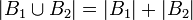$\left| B_1 \cup B_2 \right| = \left| B_1 \right| + \left| B_2 \right|$, then$dim(span(W_1 \cup W_2)) = dim(W_1) + dim(W_2)$. Q.E.D.